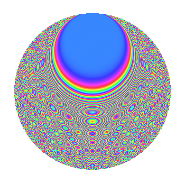# Properties

 Label 731.2.pLevel 731 Weight 2 Character orbit p Rep. character $$\chi_{731}(16,\cdot)$$ Character field $$\Q(\zeta_{14})$$ Dimension 384 Newforms 1 Sturm bound 132 Trace bound 0

# Related objects

## Defining parameters

 Level: $$N$$ = $$731 = 17 \cdot 43$$ Weight: $$k$$ = $$2$$ Character orbit: $$[\chi]$$ = 731.p (of order $$14$$ and degree $$6$$) Character conductor: $$\operatorname{cond}(\chi)$$ = $$731$$ Character field: $$\Q(\zeta_{14})$$ Newforms: $$1$$ Sturm bound: $$132$$ Trace bound: $$0$$

## Dimensions

The following table gives the dimensions of various subspaces of $$M_{2}(731, [\chi])$$.

Total New Old
Modular forms 408 408 0
Cusp forms 384 384 0
Eisenstein series 24 24 0

## Trace form

 $$384q - 12q^{2} - 72q^{4} - 8q^{8} + 62q^{9} + O(q^{10})$$ $$384q - 12q^{2} - 72q^{4} - 8q^{8} + 62q^{9} - 18q^{13} - 12q^{15} - 20q^{16} - 14q^{17} + 30q^{18} + 8q^{19} + 20q^{21} + 46q^{25} + 2q^{26} - 30q^{30} + 50q^{32} - 90q^{33} + 8q^{34} + 52q^{35} - 328q^{36} - 46q^{38} + 184q^{42} + 60q^{43} - 2q^{47} - 340q^{49} - 172q^{50} - 68q^{51} + 38q^{52} - 8q^{53} - 28q^{55} + 10q^{59} + 58q^{60} - 44q^{64} + 90q^{66} - 104q^{67} + 60q^{68} - 2q^{69} + 40q^{70} - 164q^{72} - 72q^{76} + 94q^{77} + 120q^{81} - 66q^{83} + 112q^{84} - 96q^{85} - 136q^{86} + 72q^{87} - 6q^{89} - 220q^{93} - 142q^{94} + 124q^{98} + O(q^{100})$$

## Decomposition of $$S_{2}^{\mathrm{new}}(731, [\chi])$$ into irreducible Hecke orbits

Label Dim. $$A$$ Field CM Traces $q$-expansion
$$a_2$$ $$a_3$$ $$a_5$$ $$a_7$$
731.2.p.a $$384$$ $$5.837$$ None $$-12$$ $$0$$ $$0$$ $$0$$